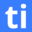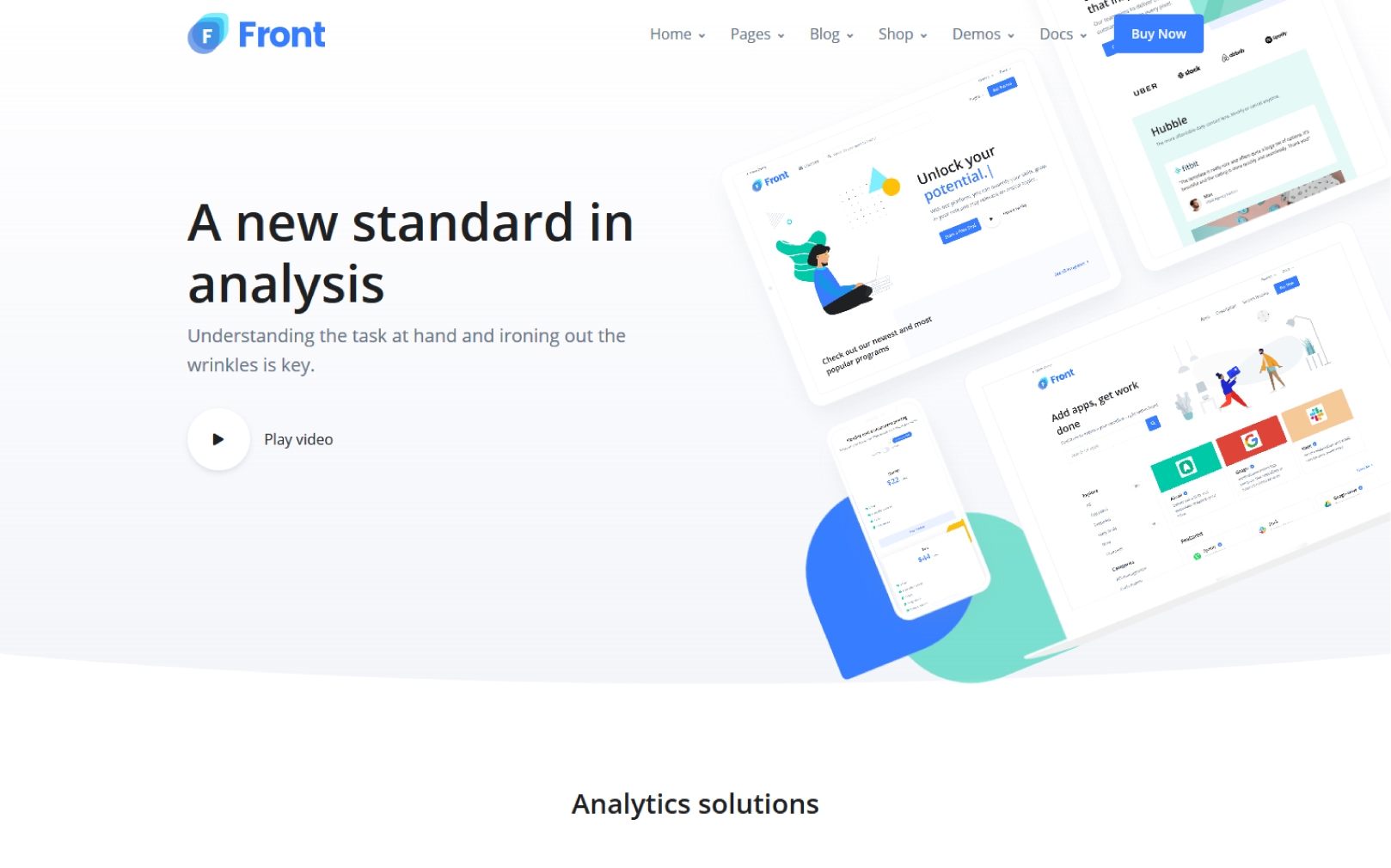# Utilize the Best Online Fundamental of Mathematics Test for Recruitment

The Fundamentals of Math (Intermediate) test is a tool designed to evaluate the mathematical skills of candidates at an intermediate level. The test covers a range of topics, including basic mathematical operations such as addition, subtraction, multiplication, and division, as well as ratios, decimals, complex fractions, and basic algebraic equations. The test consists of multiple-choice questions, problem-solving exercises, and equations to solve, among other formats. By assessing the candidate's proficiency in fundamental mathematical concepts, the test aims to determine their potential to excel in roles that require a strong foundation in mathematics, such as accounting, data analysis, or finance.Created by Testinvite

10 mins

10

Intermediate

English

## About the Fundamental of Mathematics Test (Intermediate)

Mostly used for

The Fundamentals of Math (Intermediate) test is an assessment tool that evaluates the mathematical skills and knowledge of candidates at an intermediate level. The test covers a broad range of mathematical topics, including basic operations, ratios, decimals, complex fractions, and basic algebraic equations. This test is particularly beneficial for assessing the mathematical aptitude of candidates applying for roles in accounting, finance, data analysis, and other positions that require a strong foundation in mathematics.

One of the main use case of the Fundamentals of Math (Intermediate) test is that it provides recruiters and employers with a reliable and objective way to assess the mathematical proficiency of candidates. This test enables employers to identify candidates who have a solid understanding of basic mathematical concepts and who can apply this knowledge in practical situations. By assessing candidates' skills in areas such as ratios, decimals, complex fractions, and basic algebraic equations, employers can evaluate their potential to excel in positions that require mathematical aptitude.

Moreover, the Fundamentals of Math (Intermediate) test can help recruiters and employers to screen large numbers of candidates quickly and efficiently. The test can be administered online, and its re sults can be easily and quickly analyzed, providing employers with valuable insights into candidates' mathematical proficiency. This makes it an ideal tool for recruitment processes that require a high volume of candidates to be assessed.

The Fundamentals of Math (Intermediate) test is a valuable tool for assessing the mathematical aptitude of candidates applying for roles in accounting, finance, data analysis, and other positions that require a strong foundation in mathematics. By providing recruiters and employers with an objective way to evaluate candidates' skills in areas such as basic operations, ratios, decimals, complex fractions, and basic algebraic equations, this test enables employers to identify candidates who have the potential to excel in these roles.

### Start analytical screening for hiring!

Why Testinvite talent solutions?

## Make great hiring decisions, fast.## FAQs

We recommend combining Testinvite Talent Core Skill Tests to create the best assessment solution for your recruitment process. You can add up to six tests to each assessment.

There are no extra charges or hidden fees in Testinvite. Testinvite has a transparent credit-based system that allows you to pay only for what you use. You can find pricing details here.

Testinvite safe assessment browser enables you to conduct secure assessments and protect the full integrity of online exams. You can find detailed information about how to enhance security and which security measures you need to take here.

Yes, validity and reliability are at the core of our test development methodology. We use advanced statistical methods to make sure the test has validity and reliability. You can find detailed information about our methodology here.

Fundamental Math Skills are basic numeracy skills that refer to broader cognitive abilities. The main fundamental math topics are Addition and Subtraction, Multiplication and Division, Decimals and Fractions, Ratios and Rates, Signed Numbers, Roots, Measurement, and Geometry.